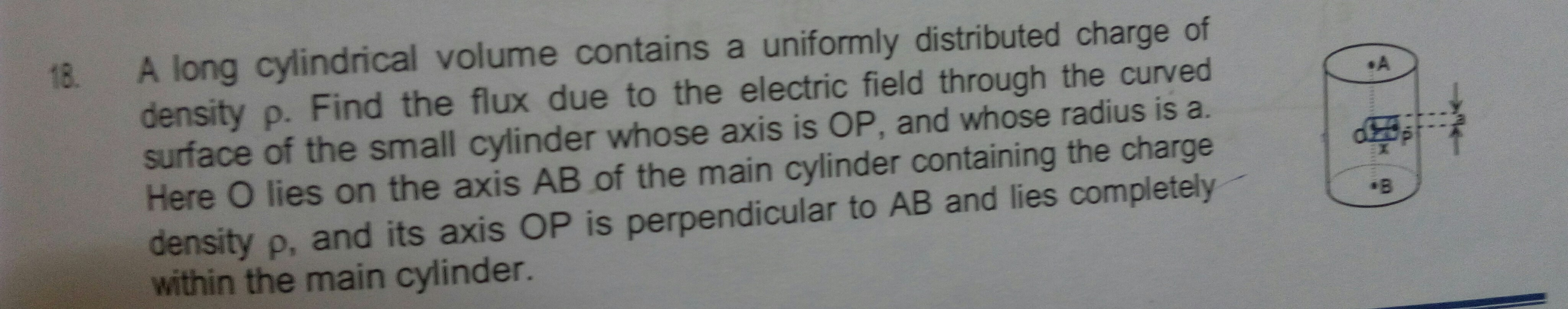Note by Ishan Dixit
4 years, 3 months ago

This discussion board is a place to discuss our Daily Challenges and the math and science related to those challenges. Explanations are more than just a solution — they should explain the steps and thinking strategies that you used to obtain the solution. Comments should further the discussion of math and science.

When posting on Brilliant:

• Use the emojis to react to an explanation, whether you're congratulating a job well done , or just really confused .
• Ask specific questions about the challenge or the steps in somebody's explanation. Well-posed questions can add a lot to the discussion, but posting "I don't understand!" doesn't help anyone.
• Try to contribute something new to the discussion, whether it is an extension, generalization or other idea related to the challenge.

MarkdownAppears as
*italics* or _italics_ italics
**bold** or __bold__ bold
- bulleted- list
• bulleted
• list
1. numbered2. list
1. numbered
2. list
Note: you must add a full line of space before and after lists for them to show up correctly
paragraph 1paragraph 2

paragraph 1

paragraph 2

[example link](https://brilliant.org)example link
> This is a quote
This is a quote
    # I indented these lines
# 4 spaces, and now they show
# up as a code block.

print "hello world"
# I indented these lines
# 4 spaces, and now they show
# up as a code block.

print "hello world"
MathAppears as
Remember to wrap math in $$ ... $$ or $ ... $ to ensure proper formatting.
2 \times 3 $2 \times 3$
2^{34} $2^{34}$
a_{i-1} $a_{i-1}$
\frac{2}{3} $\frac{2}{3}$
\sqrt{2} $\sqrt{2}$
\sum_{i=1}^3 $\sum_{i=1}^3$
\sin \theta $\sin \theta$
\boxed{123} $\boxed{123}$

Sort by:

Using gauss theorem you can easily find the dependence of field on $r$ the distance from the axis.For this use $E2πrh=\rhoπr^2h$.The net flux through the part(cylinder) is $Q/\epsilon$.Now this is equal to $\phi1$+$\phi2$.To find $\phi2$ or the flux through the circular face use the very definition of flux $\phi$=$EdScos\alpha$.And note that the field that we computed is radially outwards.An important aspect to note is that one can't use Solid Angle here as the field dependence isn't $1/r^2$.So use the trivial method.

- 4 years, 3 months ago

Answer is $px(\pi*a^2)/2\in$

- 4 years, 3 months ago

but it isnt coming from this method please provide solution

- 4 years, 3 months ago

I have provided.If it weren't small then use integration.

- 4 years, 3 months ago

Thanks got it

- 4 years, 3 months ago

One thing how does the term R gets sorted ?

- 4 years, 3 months ago

I have changed it,the variables.Now its fine I guess.

- 4 years, 3 months ago

But how would the height of small cylinder that is x will come in answer

- 4 years, 3 months ago

Note that its $r=x$ and The Radius is $R=a$.Substitute the variables now.OK?

- 4 years, 3 months ago

K

- 4 years, 3 months ago

Yes its correct.Another thing worth noting is small circular face So we can neglect the small angle $\alpha-->0$.So $\phi2$=$EπR^2$=$\rho r/2\epsilon*πR^2$.And Subtract this from $\phi$=$\rho πR^2r/\epsilon$.The answer comes to be $1/2$$\phi$

- 4 years, 3 months ago